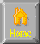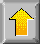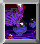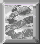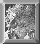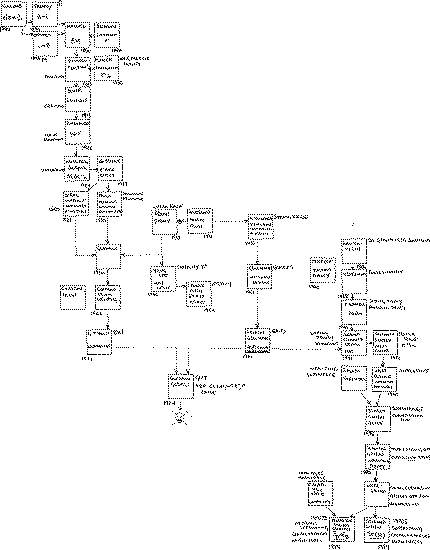```
Quantum Universe
---------------------------------------------------------------------

1. Waves vs Particles
a. Science and Experiment
b. Light and Quantum Mechanics
c. The Double Slit Experiment

2. Heisenberg and Uncertainty
a. Watching Electrons
b. Heisenberg's Uncertainty Principle
c. Uncertainty and Photography
d. Feynman's Quantum Paths

3. Schrodinger and Matter Waves
a. De Broglie's matter waves
b. Schrodinger's Equation
a. Electron and Neutron Optics

4. Atoms and Nuclei
a. Ruthurford's Nuclear Atom
b. Quantized Energy Levels
c. The Hydrogen Atom
d. Wavefunctions and Quantum Numbers

5. Quantum Tunneling
a. Barrier Penetration
b. Wave Tunneling
c. Applications of Quantum Tunneling
d. Nuclear Physics and alpha decay
e. Nuclear fusion and Nuclear fission

6. Pauli and the elements
a. Electron spin and Pauli's exclusion principle
b. The elements
c. Metals, insulators and semiconductors
d. Transistors and microelectronics

7. Death of a star
a. A failed star
b. Hydrogen burning
c. Red giants and white dwarfs
d. Neutron stars and black holes

8. Quantum co-operation and superfluids
a. Laser light
b. Bose condensation and superfluid helium
c. Superconductivity

9. Feynman rules
a. Dirac and antiparticles
b. Feynman diagrams and virtual particles
c. Zero-point motion and vacuum fluctuation
d. Hawking radiation and black holes

10. Weak photons and strong glue
a. The double-slit experiment revisited
b. The birth of particle physics
c. Weak photons and the Higgs vacuum
d. Quarks and gluons
e. Superconductors, magnetic monopoles and quark confinement

+------------+
Waves vs
Particles
+------------+
||
\/
+------------+
Uncertainty
principle
+------------+
||
\/
+------------+        +------------+
Probability          Lasers and
+-=============waves and the  ====>  superfluids
||             Schrodiner equ
||             +------------+        +------------+
||                   ||                    ||
\/                   \/                    \/
+------------+       +------------+         +------------+
Quantized energy     Quantum tunneling      Relativity  &
levels and the       and nuclear decays     quantum mechanics
Hydrogen atom
+------------+       +------------+         +------------+
||                   ||                    ||
\/                   \/                    \/
+------------+       +------------+         +------------+
Exclusion           Quantum mechanics     Fundamental forces
principle and           of                     and
the periodic table    stars                   Particles
+------------+       +------------+         +------------+

=============================================================================
1. Waves vs Particles
a. Science and Experiment
b. Light and Quantum Mechanics
1600 Newton - light = a stream of particles
1800 Thomas Young = showed light a wave motion
double slit wave interference experiemnt
Photo-electric effect  - UV light on (-) charge metal
causes it to lose its charge.
visible light has no effect on it.
Einstein 1905 light=photons, UV = high energy photons
enough energy to knock out electrons in (-) metal
c. The Double Slit Experiment
I. Bullets through double - slit, P1, P2, P12 w/ nointerference Fig1.7
I. Water Waves  through double-slit
I1+I2=H1^2+H2^2 != I12=(H1+H2)^2
I. Electron gun thin metal screen w/ slits, on phosphor screen
Fired like bullets, arrive like bullets but
interfere like water. P1=a1^2, P2=a2^2
P12=(a1+a2)^2
INC : FIG 1.11
/*---------------------------------------------------------------------*/

2. Heisenberg and Uncertainty
a. Watching Electrons
Can't measure position + momentum simultaneously.
In double slit experiment if shine light right behind
slits to find out if electron went through 1 or 2,
photon imparts enough energy so that no more interference pattern!
b. Heisenberg's Uncertainty Principle
p=mv(momemtum)
To measure position - need very short wavelength (peak-peak = min dist)
short wavelength = hi freq. E=hf (so hi energy)
(del h)*(del p) ~= h (planck's constant)

c. Uncertainty and Photography
To see a star, photon excites retinal cell/photo emulsion.
Ag+ = photo plate, photon via photoelectric absorbed e- is emitted.
So now e- absorbed by Ag+. remaining Ag+ deposited on opaque metallic silver.
CCD -> array of photon detectors in a Si chip.
Semiconductor - allow i to flow, insulator which don't allow i to flow.
Si - little en to release e- from parent atoms.

Accumulated charge / position on array = pattern of photon arrival.
d. Feynman's Quantum Paths
a=a1+a2 (for 2 slits) a=quantum amplitude
INC : fig2.8
If repeat w/ more screens added with more slits,
the quantum amplitude for an e- to travel from s to d
may be regarded as a sum over all possible paths.
If e- at lowest energy state, must constantly be in motion,
else will know both position and momentum.
e. Mathmematical Curiosity
plotting position vs time of a lowest energy e-
jiggling, sampled at finer and finer intervals,
the plot is more and more jiggly.  This is a
property of a fractal.
e.g. measuring a coast-line at varying distances.

/*---------------------------------------------------------------------*/

3. Schrodinger and Matter Waves
a. De Broglie's matter waves - connect wave+particle properties of matter
Corpuscular theory vs wave theory of photons/light
>All matter(even fundamental particles like e-)displays a wavelike nature.
Davisson and Germer demonstrated this 1929
+If all particles behave like waves why didn't observe matter waves?  don't see it
for bullets and cards?  happens @ Planck's scale
+Particle w/ momentum p & matter wave of wavelength Lambda
lambda = h / p (de broglie's relation)
+For double-slit experiment to see wavelike interference effect , the separation of
the 2 slits must be about same size as wavelength of the objects that are doing
the interfereing.  So we don't see wavelike behaviour for everyday objects.
b. Schrodinger's Equation
+Enables physicist to calculate how quantum probability waves move.
Newton->law of classical physics, Schrodinger law of motion of quantum objects.
+Conservation of energy.  e.g. roller coaster valley.  At top KE=0 PE=HI, at valley
KE=HI, PE=0 go up other side until KE=0, PE=HI. (qv Fig3.3)
+  E = K + V ( Total energy = kinetic energy + potential energy) eq1
+  p = mv (momentum = mass x velocity) eq2
+  K = (p^2) / (2m) eq3 (plug eq3 into eq1 -> E = (p^2)/(2m) + V )
+  Can set up a roller coaster for electrons fig 3.4;
o ->  ___(-)___ -> ___(+)___ -> ___(-)___  (charged plates)
++  Schrodinger's equation
For the motion of a particle with total energy E, moving in one dimension,x, in
a region in which there is a potential, V schrodinger's equation reads
E(psi) = - (h/2pi)^2 /(2m)  d^2(psi)/dx^2 + V (psi)
It is the psi = probability amplitudes
m   = mass of particle
h   = planck's constant

a. Electron and Neutron Optics
+Matter waves = wavelike behavior of particles.
+Electron Microscope()
+Linear Accelerators () , quarks have partial charges.  Elementary building blocks
of matter (Murray Gell-Mann - George Zweig).
+Wavelike properties can be demonstrated for several other particles.  Neutrons.
Neutron double slit experiment ()

/*---------------------------------------------------------------------*/
Atoms and Nuclei
a. Ruthurford's Nuclear Atom
+1911 Rutherford showed most of mass in atom in nucleus. (alpha particle())
+  Radioactive decay ()
Alpha rays = helium atoms w/ 2 lost electrons
Beta rays  = electrons
Gamma rays = high energy photons
+  Nucleus = Protons + Neutrons (2000xweight of e-)
+  strong force holds protons together in nucleus.
(allow only certain numbers of neutrons + protons to combine to form stable
nuclei)
+  p+ (normal hydrogen) ; n&p+ (deuterium:D) ; n&n&p+ (tritium-T)
+  p+&p+&n (Helium 3 3^He) ; p+&p+&n&n (Normal helium 4^He)
+  Isotopes of hydrogen used in nuclear reactions of stars , nuclear weapons.
Isotopes, decay to more stable elements.
+  Ruthuerford introduce (now outdated) orbital electron-nucleus model. #list fail
+  Bohr shows stable orbits.
+  Johann Jakob Balmer - line spectra()
+  Bohr's allowed electron orbits explained balmer's formula
line spectra conformed to formula
lambda = ((364.5) n^2)/ (n^2  -4 ) where n=3,4,5,6
b. Quantized Energy Levels
+  Electrons in motion round the nucleus only allowed in certain orbits.
+  Orbits unstable since electrons can radiate away energy
+  Each orbit corresponds to a specific energy for the electron
+  Energy is "quantized" = stable orbits.
+  how electron orbitals ()
+  qv INC : fig 4.9
+  Note lowest ground energy state is not zero (sphere).  Heisenberg's uncertainty
principle requires a certain minimum energy.   must forever be jiggling.
+  Probability amplitude for electron in the box vanishes not only at the ends
but at nodes.  Nodes = places where no movement of string, but for e-
nodes = places where there is no chance of finding the electron.
+  Probability of finding the electron at different positions wi / the box =
square of quantum probability amplitude (psi^2).
+  Allowed energies quantized, but probability of finding the electron varies with
position wi/ the box is different for different electron energies.
+  Quantum levels labeled (n=1=ground state...)
+  qv fig 4.10, 4.11 ; electron in 2d box has same solutions,
but excitation in x or y.  Label wavefunctions 2x and 2y.
2x and 2y (p orbitals) more than 1 state w/ same energy is degenerate.
c. The Hydrogen Atom
+  Hydrogen = 1 proton , 1 e-
+  e- attracted to p+, classically electron lower its energy
until sitting on top of p+
+  quantum mechanics Heisenberg's uncertainty principle prevents this.
+  Planck's formula E = hf (energy of photon = frequency x planck))
+  left to itself e- occupies ground state.  If e- excited
jumps to high energy state, decays to ground state.
gives off photon w/ energy : Ephoton = Einitial - Efinal
+   c = f lambda (velocity of light = frequency x wavelength)
+   Schrodinger's result same as balmer and bohr ()
+   So photons must be absorbed with energy equal to a energy level difference!
+   qv INC : Fig 4.12
+   Energy differences from ground state to any excited state are in ultra-violet
(appendix1) . So visible light can pass through many gases unabsorbed, since
atoms require a much larger photon energy for excitation from ground state.
Reason why most gases are transparent to visible light.
+   qv Fig 4.13
+   Emission spectrum()
d. Wavefunctions and Quantum Numbers
+ Ground state (sphere) energy quantum # n=1.
probability of finding an e- is proportional to psi^2.
this probability density doesn't change w/ time (so no acceleration
so no light given off, so no spiraling in of e- toward nucleus)
+ Excited energy level (dilobe 2Px 2Py 2Pz)
there is degenercy = i.e. 2Px looks like 2Py has same energy
different angular momentum.
+  L = rp (angular momentum = length of string x ordinary momentum)
angular momentum is conserved.
so if length r is decreased p must increase (ball on string)
+  Quantum mechanical angular momentum is quantized (like energy)
Angular momentum only allowed to take values whole units of planck constant/2pi.
+   n=1 M=0 L=0             sphere           S (sharp)
n=2 L=0 M=0             sphere           P (principle)
n=2 L=1 M=+1,0,-1       dilobe           P
n=3 L=0 M=0             nested sphere    D (diffuse)
n=3 L=1 M=+1,0,-1       quad lobe        D
n=3 L=2 M=+2,+1,0,-1,-2 dilobe,quadlobe  D
n=4 ...                                  F (fundamental)
+  understanding of these 3 quantum numbers provides nearly
entire understanding of mendeleev's periodic table of elements.

/*---------------------------------------------------------------------*/

Quantum Tunneling
a. Barrier Penetration
+ Quantum objects can tunnel through potential energy barriers that classical
objects are forbidden to penetrate. qv fig 5.1
+ Barrier penetration / quantum tunnelling basis for
Tunnel diode and Josephson junction.
+ How ? (can use schrodinger eqn) or Heisenberg's uncertainty principle.
Position + Momentum. Also another
(del E)(del t) ~= h  (Time + Energy roughly = planck's constant)
Can never change total amount of energy, but if
time uncertainty is del t.  We cannot know energy better than
uncertainty delE = h/delt.  So we can borrow an energy
del E if we repay it wi/ time delt = h/del E.
If barrier to too high or too wide tunnelling becomes unlikely.
and electrons will be reflected.
tunneling = general property of wave motion.
surprising w/ de Broglie => all quantum particles have wavelike properties.
b. Wave Tunneling
+ refraction()
+ if angle increased = total internal reflection()  fibre optics
+ no light escapes if light @ angle > critical angle, but a standing
wave (evanescent wave()  ) is created
+ Tunneling happens if we bring up another block of glass parallel to the
first.  As we bring the 2 blocks together the evanescent wave penetrates
the second block and light appears.  This is because the
amplitude of the standing wave in the forbidden air gap has not had
time to decay away.  "frustrated total internal reflection"
+ qv Fig 5.5 shows ripple tank photograph of barrier penetration w/ water waves.
c. Applications of Quantum Tunneling
+ Devices based on ability of quantum particles to tunnel through
barriers. electrons, alpha particles , pairs of electrons.
+ Field emission microscope()
+ Field ion microscope()
+ Scanning tunnelling microscope()
+ Tunnel diode()
d. Nuclear Physics and alpha decay
+Radioactive decay of uranium puzzling.
strong force and p+ to p+ attraction = 30MeV potential well
keeps p+ and n inside nucleus.  though justling inside.
some combine into alpha particle.
alpha particle @ 4MeV thrown out. But normally takes 30MeV to escape
tunnel out. Borrow 26MeV return it in time delT.
+INC : See fig5.9 George Gamow explained 1928
+ Cockcroft & Walton also able to shoot alpha particles and
penetrate nucleus.  Normally require many MeV to
surmount electric repulsive barrier surrounding nucleus
but penetrated by tunneling w/ <1MeV.
1|_|   7|       4|_|   4|_|
1| | + 3|_i  -> 2| |e+ 2| |e
proton mass + lithium mass + kinetic en of proton)
=> 2x(helium mass + kinetic en of helium)
resulting 8MeV kinetic en(K) of He much higher than proton K
+ E = mc^2
e. Nuclear fusion and Nuclear fission
+ deuteron binding energy ()
+ Binding energy = strong force energy which holds nucleons together.
+ plot average binding energy per nucleon for various elements. Fig 5.13
+ two ways of releasing energy from nucleus.  Fusion/Fission.
binding en released in Fussion/fission appears as kinetic energy of
final particles.
+ D-T (deuterium-Tritium fusion ) reaction ()
+ Tough to get a stable sustained reaction.
why? because of electrical repulsive coulomb barrier.
+ cheap fusion power = heat up initial constituents to high temp
so enough kinetic energy in collision of plasma
containment of hot plasma = prob.
stars use cold fusion, but temp in stars < coulomb barrier!
use quantum tunnelling through potential barrier.
+ INC : Fig 5.15
+ Why are some nuclei stable , others radioactive? (alpha,beta,gam decay)
+ strong force holding nucleons together
coulomb electrical repulsion of protons trying to break nucleus apart.
+ INC : Fig 5.16
+ In heavy nucleus balance of coulomb repulsion w/ attractive strong
force  delicate. can hit U235 w/ n to split into Rb93+C141+n+Energy
+ Fission can be thought as tunnelling process.
energy of fissioning nucleus   like Fig 5.1
2 valleys/minima of energy (1 lower than other)
quantum mechanically a particle in upper valley has chance
to tunnel to true lowest energy
+ Can use chain reaction (resultant neutrons from rx in fig5.16
start process over again)  - atom bomb,
or nuclear power plant control reaction.
+ Dating w/ radioactivity()

/*---------------------------------------------------------------------*/

6. Pauli and the elements
a. Electron spin and Pauli's exclusion principle
+ Dimitri Mendeleev periodic table of elements.
+ Wolfgang Pauli - exclusion principle.
+ loop of i induces B field.
+ So w/ Bohr e- orbitals have magnetic properties
+ Zeeman Effect ()  -split spectrum
+ Uhlenbeck/Goudsmit-angular momentum of e- & spin angular momentum
e- about its own axis.
+ ch4- angular momentum quantized, so e- can spin clockwise or !clockwise.
> Pauli exclusion principle() - only 1 e- allowed in each quantum state.
+ All fermions obey pauli exclusion principle.
so "matter" cannot be compressed but must occupy a certain space.
+ "Radiation-like" particles Bosons prefer to be all together in
lowest energy state.
b. The elements
+ ch4- Schrodingers eq led to Bohr's quantized energy levels
+ Atom w/ Z protons fills Z electrons in s-p-d-f orbitals
+ atoms w/ full orbitals are inert(He,Ne,Ar).
+ atoms which can share e- to make common stable orbital (covalent bond)
(e.g. Li2,H2) are stable/inert.
c. Metals, insulators and semiconductors
+ Metals/Conductors have conduction e- able to move /carry current
w/ electrical potential applied
Insulators have no conduction electrons (no current flow w/ applied V)
Semiconductor have some conduction electrons
+ Crystalline solids vs amorphous solids. Matr	x affects allowed en lvl
+ Metals can be thought of as extreme case of covalent binding in which
all conduction electrons shared by all atoms in the metal
+ If apply potential, electrons can gain energy and be accelerated
in direction of potential by jumping to empty higher energy levels.
+ Felix Bloch quantum mechanical band structure of solids.
+ INC : Fig 6.14
+ If Energy gap big, e- can't jump = insulator; metal can easily jump...
d. Transistors and microelectronics
+ Doping - n-type - dope w/ element has left over e- which can conduct
p-type - dope w/ boron leave empty state allow conductivity
+ p-n junction allow flow of e- from 1 to another -> switch
+ p-n-p , Mosfet,
+ IC - layers of insulating , conducting, rectifying , amplfying materials
electrical functions by cutting out areas of various layers.
+ MOSFET - metal-oxide-semiconductor field effect transistor.

/*---------------------------------------------------------------------*/
Death of a Star

a. A failed star
+ Quantum mechanics and exclusion principle provide basis
for understanding of all types of matter/periodic table.
+Quantum mechanics + exclusion principle key to stellar evolution.
+jupiter = star did not quite make it ()
+Hi pressure, but in star e- and p+ squeezed apart,
so gravity overcomes electrical attraction, result is
soup of e- and p+ = plasma
+As P inc, e- and p+ move faster, plasma heats up.  This thermal motion
provides pressure to opose gravitational collapse.
+Enough dense, nuclear reactoins take place

b. Hydrogen burning
+Sir Arthur eddington, show temperature in star
too low for p+ to overcome repulsive energy barrier
and get together so nuclear rx .
+w/ Gamow's quantum tunnelling, Atkinson, Houtermans
p+ can penetrate atomic nucleus even if energy inadequate.
tunnelling.  Light nuclei = trap for p+, when 4p+
captured, alpha particle formed. this ejected from nucleus liberating
large nuclear binding energy from fusion process in which 4hydrogen
nuclei converted to He.
+Fusion like bomb, why not explode?  burns fuel much slower rate.
Bomb->deuterium-tritium fusion uses rapid strong nuclear interaction
Star->use nuclear beta decay reaction-weak interaction, slow
+Beta radioactivity() w/ weak interaction
+Beta decay process : p -> n + e+ + v
can occur is new nucleus produced by decay is more tightly bound than
original nucleus.  Since according to uncertainty principle system
can borrow extra energy to make decay possible if the system will
have reached a state w/ lower energy. key!
+stellar quantum tunnelling ()
+Proton-Proton cycle.  Main source of sun's energy.
+Some stars Bethe's mechanism()
Bethe's carbon cycle
use carbon nuclei as catalyst to cook helium.

c. Red giants and white dwarfs
+H -> He in stars. Collapse ()
+Core of red giant collapse more massive, more He.
e- crowd until Pauli doesn't allow e- w/ same quantum
# to occupy same volume.
Minimum volume from de Broglie wavelength.
Also similar Pauli principle effect for p+ and neutrons.
+White dwarf ()

d. Neutron stars and black holes
+Neutron Star () & Chandrasekar limit ()
+ Pulsar = rotating neutron star.
+ Black hole()

```
CONTINUED....